Gain

Find the gain whenever the output power is 21x the input power.

Result

g =  13.22 dB

Solution:Leave us a comment of example and its solution (i.e. if it is still somewhat unclear...):Be the first to comment!Next similar examples:

1. SequenceFind the common ratio of the sequence -3, -1.5, -0.75, -0.375, -0.1875. Ratio write as decimal number rounded to tenth.
2. Decibel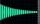A circuit has an input power of 58 mW. Its output power is 6 mW. What is the loss in decibels?
3. Seating rules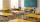In a class are 24 seats but in 7.B class are only 18 students. How many ways can student seat? (The class has 12 benches. A bench is for a pair of students.) Result (large number) logarithm and thus write down as powers of 10.
4. Train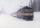The train passes part of the line for 95 minutes at speed 75 km/h. What speed would have to go in order to shorten the driving time of 20 minutes?
5. Virus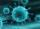We have a virus that lives one hour. Every half hour produce two child viruses. What will be the living population of the virus after 3.5 hours?
6. LogarithmDetermine the number whose decimal logarithm is -3.8.
7. Intercept with axisF(x)=log(x+4)-2, what is the x intercept
8. Theorem proveWe want to prove the sentence: If the natural number n is divisible by six, then n is divisible by three. From what assumption we started?
9. Complex number coordinatesWhich coordinates show the location of -2+3i
10. CalculationHow much is sum of square root of six and the square root of 225?
11. AS sequenceIn an arithmetic sequence is given the difference d = -3 and a71 = 455. a) Determine the value of a62 b) Determine the sum of 71 members.
12. SequenceBetween numbers 1 and 53 insert n members of the arithmetic sequence that its sum is 702.
13. DiscriminantDetermine the discriminant of the equation: ?
14. TrinityHow many different triads can be selected from the group 43 students?
15. RootsDetermine the quadratic equation absolute coefficient q, that the equation has a real double root and the root x calculate: ?
16. Scalar product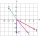Calculate the scalar product of two vectors: (2.5) (-1, -4)
17. Linsys2Solve two equations with two unknowns: 400x+120y=147.2 350x+200y=144